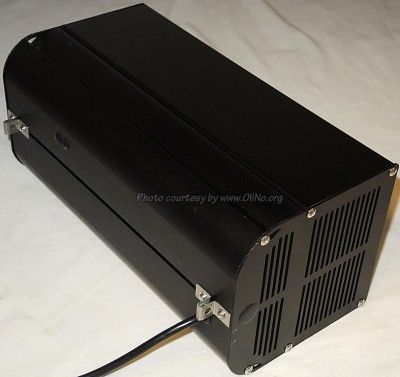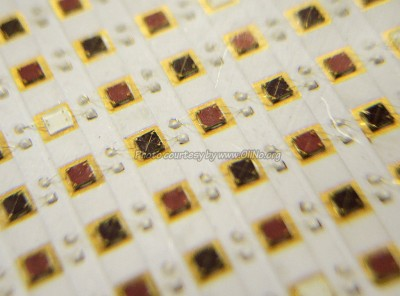# OliNo

Renewable Energy

## VariPar Distribution BV – RA 350 COB LED plant grow light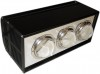presents an LED plant grow light. The measurements from OliNo show that the lamp consumes 321.0 W and has a luminous flux of 7523 lm, or 348 micromol/s.

This article shows the measurement results. Many parameters are also found in the Eulumdat file.

See this overview for a comparison with other light bulbs.

### Summary measurement data

parameter meas. result remark
Luminous intensity Iv 4941.3 Cd Measured straight underneath the lamp.
Illuminance modulation index 2 % Measured with a light sensor looking at the lamp (angle not defined). Is a measure for the amount of flickering.
Beam angle 81 deg 81 deg is the beam angle for all C-planes since the lamp is symmetrical along its 1st axis.
Power Factor 0.99 An electrical load with this power factor means that for every 1 kWh net energy consumed, there has been 0.17 kVAhr for reactive energy.
THD 4 % Total Harmonic Distortion.
Max inrush current 37.013 A This current has been found at a voltage start angle of 90 degrees.
Luminous flux 7523 lm Measured with photogoniometer, calculation done as described in LM79-08.
Luminous efficacy 23 lm/W
Coordinates chromaticity diagram x=0.4827 en y=0.1952
Fitting 230V This lamp is connected directly to the grid voltage.
PAR-value 177.2 uMol/s/m2 The number of photons seen by an average plant when it is lit by the light of this light bulb. Value valid at 1 m distance from light bulb.
PAR-photon efficacy 0.8 uMol/s/We The total emitted number of photons by this light, divided by its consumption in W. It indicates a kind of efficacy in generating photons.
Photon current 347.8 uMol/s The total number of photons in the light of this lamp.
S/P ratio 1.4 This factor indicates the amount of times more efficient the light of this light bulb is perceived under scotopic circumstances (low environmental light level).
L x W x H external dimensions 360 mm x 160 mm x 180 mm External dimensions of the lamp.
L x W luminous area 280 mm x 80 mm Dimensions of the luminous area (used in Eulumdat file). It is the area of the smallest rectangle containing the three LEDs.
General remarks The ambient temperature during the whole set of illuminance measurements was 27.2 – 28.3 deg C.The temperature of the housing gets maximally about 26 degrees hotter than ambient temperature.Warm up effect: During the warmup time the illuminance varies during 31 minutes and decreases with 22 %.
During the warmup time the power doesn’t vary significantly (< 5 %).
The variation in efficacy (calculated as indication by simplydividing the illuminance by the power) during the warming up is -18 %. A very high negative value indicates a significant decrease for instance due to heating up of the lamp (decrease of lifetime).Voltage dependency: There is no (significant) dependency of the illuminance when the power voltage varies between 200 – 250 V AC.
There is no (significant) dependency of the consumed power when the power voltage varies between 200 – 250 V AC.At the end of the article an additional photo.
Eff-variation -18 % This is the variation in efficacy (calculated as indication by simply dividing the illuminance by the power) during the warming up. A very high negative value indicates a significant decrease for instance due to heating up of the lamp (decrease of lifetime).
Dimmable yes Info from manufacturer.

Biologic effect factor
1.004 According to pre-norm DIN V 5031-100:2009-06.
Blue Light Hazard risk group 1 0=exempt, 1=low, 2 = moderate, 3=high risk.
form factor pendant
Eulumdat fileRight click on icon and save the file.
Eulumdat file (with micromol/s instead of lm)Right click on icon and save the file.

### Overview table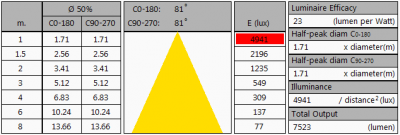The overview table is explained on the OliNo website.

Please note that this overview table makes use of calculations, use this data with care as explained on the OliNo site. E (lux) values are not accurate, when within 5 x 291 mm (maximal luminous size, eventually diagonally measured)= 1455 mm. Within this distance from the lamp (data given in red), the measured lux values will be less than the computed values in this overview as the measurements are then within the near field of the lamp.

### EU 2013 Energy label classification

Important for the energy classification are the corrected rated power and the useful luminous flux.
The measured rated power is 321.0 W and might need to be corrected. The correction is dependent from the lamp type and whether or not the lamp control gear is included or not. The choice for this lamp is the following classification: Lamps with own control gear (external or internal). As a result the corrected rated power becomes: 321.0 W.
The luminous flux measured is 7523 lm. The classification of this lamp needed to determine the useful flux is: Other directional lamps. Then the useful flux becomes 6749 lm. Now a reference power can be calculated.

The energy efficiency coefficient is P_corr / P_ref = 0.65.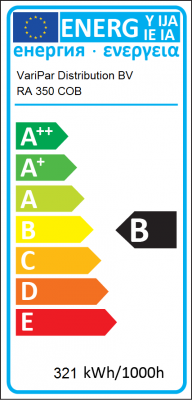EU energy label for this lampZip file with 6 EU energy labels of this lamp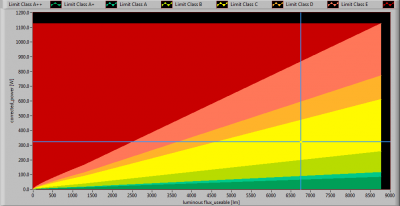The lamp’s performance in the lumen-Watt field, with the energy efficacy fields indicated.

### Eulumdat light diagram

This light diagram below comes from the program Qlumedit, that extracts these diagrams from an Eulumdat file. This light diagram is explained on the OliNo site.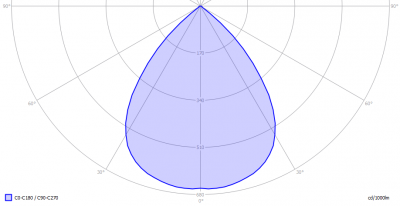The light diagram giving the radiation pattern.

The light diagram indicates the beam in the C0-C180 plane and in the plane perpendicular to that, the C90-C270 plane. These beams are equal as the lamp has symmetry over its first axis (the vertical axis).

The light distribution when micromol/s are considered: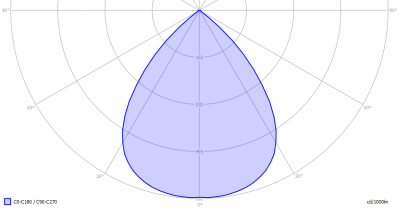### Illuminance Ev at 1 m distance, or luminous intensity Iv

Herewith the plot of the averaged luminous intensity Iv as a function of the inclination angle with the light bulb.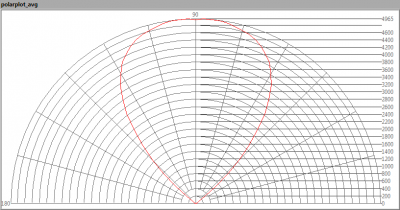The radiation pattern of the light bulb.

This radiation pattern is the average of the light output of the light diagram given earlier. Also, in this graph the luminous intensity is given in Cd.

These averaged values are used (later) to compute the lumen output.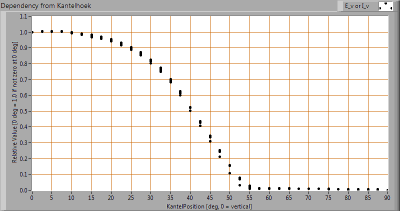Intensity data of every measured turn angle at each inclination angle.

This plot shows per inclination angle the intensity measurement results for each turn angle at that inclination angle. There normally are differences in illuminance values for different turn angles. However for further calculations the averaged values will be used.

When using the average values per inclination angle, the beam angle can be computed, being 81 deg for the C0-C180 plane and 81 deg for the C90-C270 plane.

### Luminous flux

With the averaged illuminance data at 1 m distance, taken from the graph showing the averaged radiation pattern, it is possible to compute the luminous flux.

The result of this computation for this light spot is a luminous flux of 7523 lm.

### Luminous efficacy

The luminous flux being 7523 lm, and the consumed power of the lamp being 321.0 Watt, results in a luminous efficacy of 23 lm/Watt.

### Electrical properties

The power factor is 0.99. An electrical load with this power factor means that for every 1 kWh net energy consumed, there has been 0.17 kVAhr for reactive energy.

 Lamp voltage 229.83 V Lamp current 1.418 A Power P 321.0 W Apparent power S 325.8 VA Power factor 0.99

Of this lamp the voltage across and the resulting current through it are measured and graphed. See the UI acquisition on the OliNo site how this is obtained.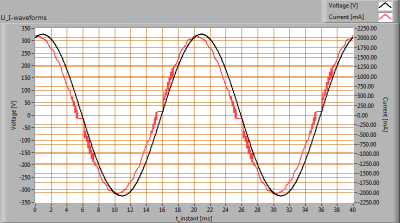Voltage across and current through the lightbulb

This current waveform has been checked on requirements posed by the norm IEC 61000-3-2:2006 (including up to A2:2009). See also the IEC 61000-3-2:2006 explanation on the OliNo website.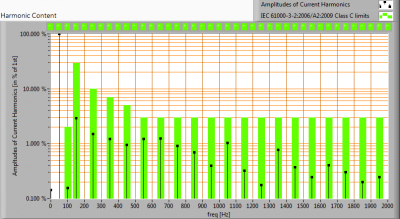Harmonics in the current waveform and checked against IEC61000-3-2:2006 and A2:2009

When the consumed power is 25 W there are limits for the harmonics and those are fulfilled.

The Total Harmonic Distortion of the current is computed and its value is 4 %.

### Inrush current

The inrush current has been measured for different voltage start angles; from 0 – 170 degrees with a 10 degrees step. The current- and voltage values have been acquires at a sample speed of 39.9 kS/s. Then this data has been fed into a second order 2kHz low pass Butterworth filter. This removes the current spikes that do not represent relevant values.
The lamp was two minutes off before every inrush current measurement was made.

 Test voltage 230.0 V Frequency of the voltage 50.0 Hz Maximum inrush current 37.013 A This current has been found at a voltage start angle of 90 degrees. Pulse width of max inrush current 9.5E-4 s This is the time that the pulse is higher than 66 % of the max inrush current. Minimal inrush current 4.176 A This current has been found at a voltage start angle of 170 degrees. I^2 x t after 10 ms at 0 deg voltage start angle 6.205E-1 A This is the I^2 t value when a zero crossing detector is used to start the voltage from 0 degrees.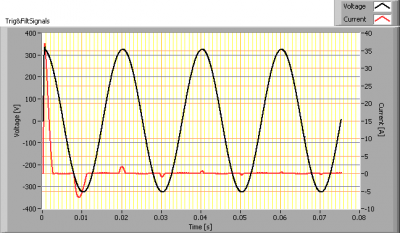Inrush current found at worst-case voltage start angle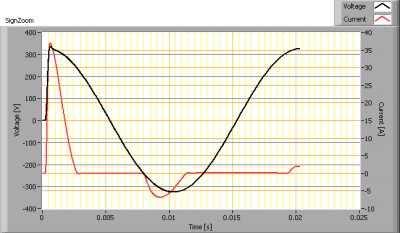First cycle of the maximum inrush current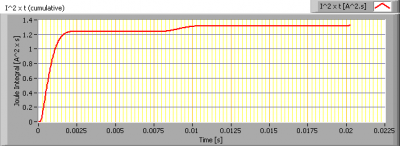The energy I2t during the first 10 ms of the first current cycle

### Temperature measurements lamp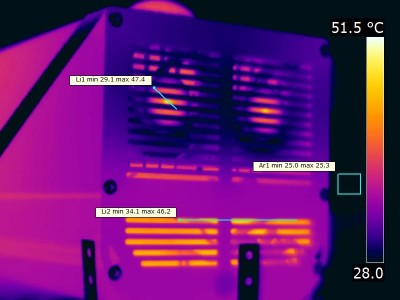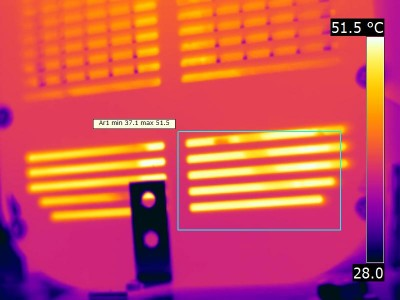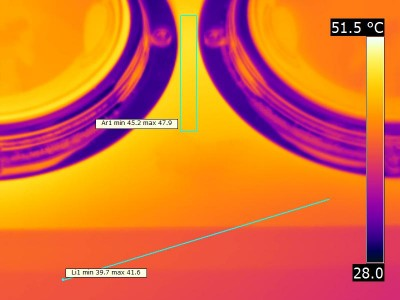Temperature image(s).

 status lamp 2 hours on ambient temperature 25.5 deg C reflected background temperature 25.5 deg C camera Flir T335 emissivity 0.95 measurement distance 0.5 m IFOVgeometric 0.136 mm per 0.1 m distance NETD (thermal sensitivity) 50 mK

### Spectral power distribution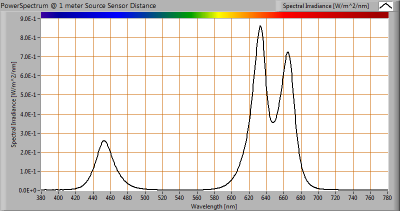The spectral power distribution of this light bulb, energies on y-axis valid at 1 m distance.

### PAR value and PAR spectrum

To make a statement how well the light of this light bulb is for growing plants, the PAR-area needs to be determined. See the explanation about PAR on the OliNo website how this all is determined and the explanation of the graph.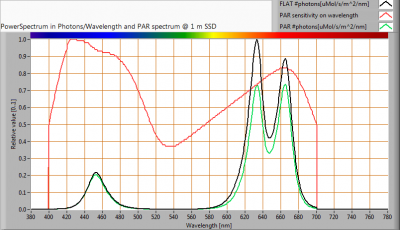The photon spectrum, then the sensitivity curve and as result the final PAR spectrum of the light of this light bulb

parameter value unit
PAR number 177.2 uMol/s/m^2
PAR photon current 269.8 uMol/s
PAR photon efficacy 0.8 uMol/s/W

The PAR efficiency is 78 % (valid for the PAR wave length range of 400 – 700 nm). This is the maximum percentage of the total of photons in the light that is effectively used by the average plant (since the plant might not take 100 % of the photons at the frequency where its relative sensitivity is 100 %).

### S/P ratio

The S/P ratio and measurement is explained on the OliNo website. Here the results are given.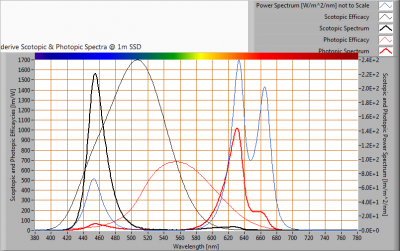The power spectrum, sensitivity curves and resulting scotopic and photopic spectra (spectra energy content defined at 1 m distance).

The S/P ratio of the light coming from this lamp is 1.4.

More info on S/P ratio can be found on the OliNo website.

### Chromaticity diagram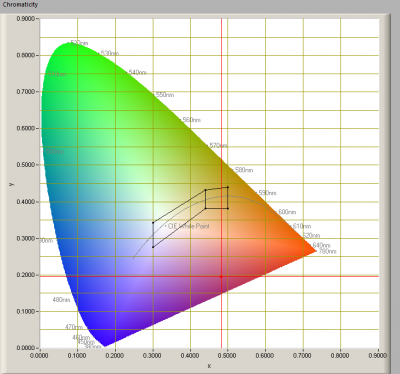The chromaticity space and the position of the lamp’s color coordinates in it.

The point of the light in this diagram is outside the area indicated with class A/B. This area indicates an area for signal lamps, see also the article on signal lamps and color areas on the OliNo website.

The color coordinates are x=0.4827 and y=0.1952.

### Voltage dependency

The dependency of a number of lamp parameters on the lamp voltage is determined. For this, the lamp voltage has been varied and its effect on the following light bulb parameters measured: illuminance E_v [lx], the lamppower P [W] and the luminous efficacy [lm/W] (this latter is estimated here by dividing the found E_v value by P).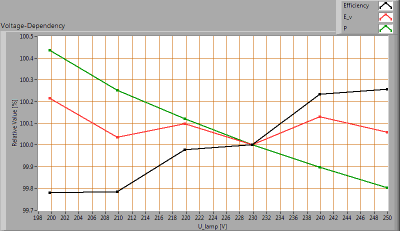Lamp voltage dependencies of certain light bulb parameters

There is no (significant) dependency of the illuminance when the power voltage varies between 200 – 250 V AC.
There is no (significant) dependency of the consumed power when the power voltage varies between 200 – 250 V AC.

When the voltage varies abruptly with + or – 5 V AC then this results in a variation of the illuminance of maximally 0.1 %. This difference in illuminance is not visible (when it occurs abruptly).

### Warm up effects

After switch on of a cold lamp, the effect of heating up of the lamp is measured on illuminance E_v [lx], the lamppower P [W] and the luminous efficacy [lm/W].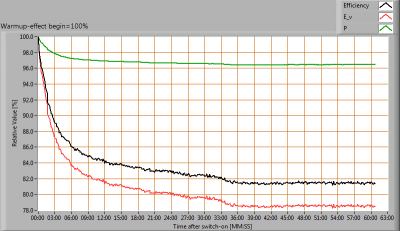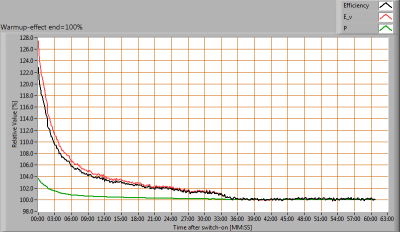Effect of warming up on different light bulb parameters. In the first graph the 100 % level is put at begin, and in the last graph the 100 % level is put at the end.

During the warmup time the illuminance varies during 31 minutes and decreases with 22 %.
During the warmup time the power doesn’t vary significantly ( 5 %).
The variation in efficacy (calculated as indication by simplydividing the illuminance by the power) during the warming up is -18 %. A very high negative value indicates a significant decrease for instance due to heating up of the lamp (decrease of lifetime).

### Measure of flickering

An analysis is done on the measure of flickering of the light output by this light bulb. See the article on flickering on OliNo site for more information.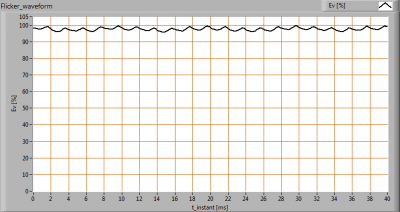The measure of fast illuminance variation of the light of the light bulb

parameter value unit
Flicker frequency 499.1 Hz
Illuminance modulation index 2 %

The illuminance modulation index is computed as: (max_Ev – min_Ev) / (max_Ev + min_Ev).

### Biologic effect

The biologic effect shows the level of impact the light of this lamp can have on the day-night rhythm of human beings (as well as the suppression of melatonin production). See also the explanatory article on biologic effect on OliNo.
The important parameters (according to prenorm DIN V 5031-100:2009-06):

 biologic effect factor 1.004 kbiol trans (25 years) 1 kbiol trans (50 years) 0.694 kbiol trans (75 years) 0.382 kpupil(25 years) 1 kpupil(50 years) 0.74 kpupil(75 years) 0.519

### Blue Light Hazard

The amount of blue light and the harm it can cause on the retina has been determined. Herewith the results.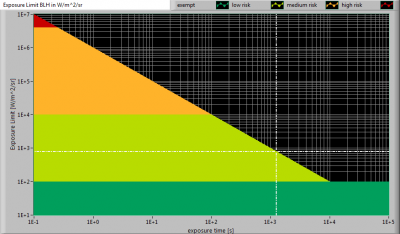The level of blue light of this lamp related to the exposure limit and the different classification areas.

 L_lum0 [mm] 50 Dimension of brightest part of lamp in C0-C180 direction. L_lum90 [mm] 150 Dimension of brightest part of lamp in C90-C270 direction. SSD_500lx [mm] 3144 Calculated distance where Ev = 500 lux. This computation is valid when it is in the far field of the lamp. Note: if this value 200 mm then the distance of 200 mm is taken as proposed in the norm IEC 62471:2006. Start of far field [mm] 791 Minimum distance at which the lamp can be seen as apoint source. In this area the Ev is linearly dependent from (1/distance)2. 300-350 nm values stuffed with 0s yes In the event OliNo has measured with a SpB1211 spectrometer without UV option then the irradiance data of 300-349 nm is missing. For lamps where there is already no energy content near 350 nm, the values 300-349 can also be set at zero then. alphaC0-C180 [rad] 0.016 (Apparent) source angle in C0-C180 direction. alphaC90-C270 [rad] 0.048 (Apparent) source angle in C90-C270 direction. alphaAVG [rad] 0.032 Average (apparent) source angle. If average >= 0.011 rad then the exposure limit is computed with radiance Lb. Otherwise with irradiance Eb. Exposure value [W/m^2/sr] 7.91E+2 Blue Light Hazard value for this lamp, measured straight underneath the lamp. Computation is referenced to Lb. Blue Light Hazard risk group 1 0=exempt, 1=low, 2 = moderate, 3=high risk.

### Extra